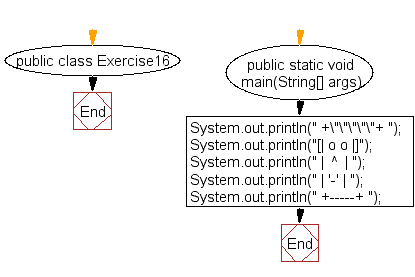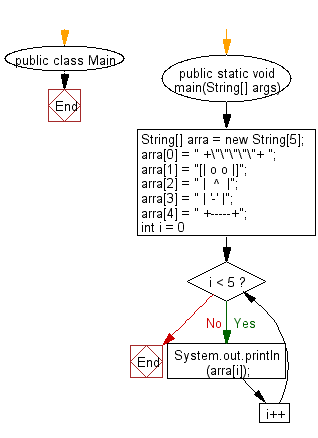﻿ Java exercises: Print a face - w3resource# Java Exercises: Print a face

## Java Basic: Exercise-16 with Solution

Write a Java program to print a face.

Pictorial Presentation:Sample Solution:

Java Code:

``````
public class Exercise16 {
public static void main(String[] args)
{
System.out.println(" +\"\"\"\"\"+ ");
System.out.println("[| o o |]");
System.out.println(" |  ^  | ");
System.out.println(" | '-' | ");
System.out.println(" +-----+ ");
}
}
```
```

Sample Output:

``` +"""""+
[| o o |]
|  ^  |
| '-' |
+-----+
```

Flowchart:Sample Solution (using array):

Java Code:

``````public class Main {

public static void main(String[] args) {

String[] arra = new String;

arra = " +\"\"\"\"\"+ ";
arra = "[| o o |]";
arra = " |  ^  |";
arra = " | '-' |";
arra = " +-----+";

for (int i = 0; i < 5; i++) {
System.out.println(arra[i]);
}

}

}
```
```

Flowchart:Java Code Editor:

What is the difficulty level of this exercise?

Test your Programming skills with w3resource's quiz.

﻿

## Java: Tips of the Day

getEnumMap

Converts to enum to Map where key is the name and value is Enum itself.

```public static <E extends Enum<E>> Map<String, E> getEnumMap(final Class<E> enumClass) {
return Arrays.stream(enumClass.getEnumConstants())
.collect(Collectors.toMap(Enum::name, Function.identity()));
}
```

Ref: https://bit.ly/3xXcFZt